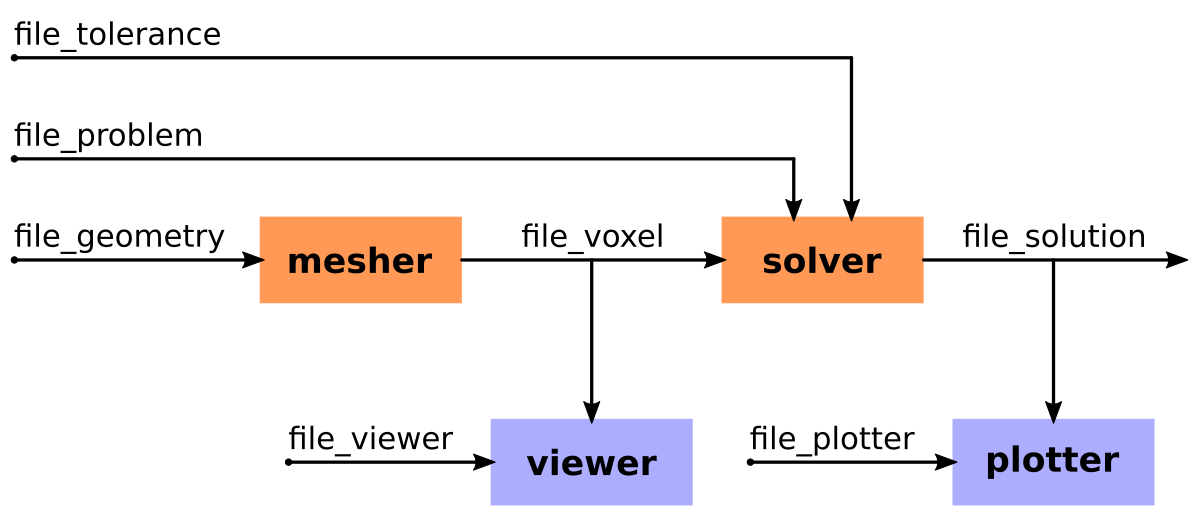# Workflow

## PyPEEC Tools

• mesher

• Create the voxel structure

• Import the geometry from voxel files

• Import the geometry from STL files

• Create the geometry with stacked PNG files

• Draw the geometry with stacked 2D vector shapes

• Assign different domain names to the voxels

• Resampling (refine) the voxel structure

• Construct the graph of the structure and detect connected components

• viewer

• Visualization of the different domains composing the voxel structure

• Visualization of the connected components composing the voxel structure

• Comparison of the voxelized and original geometries

• solver

• Computation of the incidence matrix

• Computation of the Green and coupling tensors

• Computation of the resistance, inductance, and potential matrices

• Computation of the electric-magnetic coupling matrices

• Creation of the equation system

• Extraction of a sparse pre-conditioner

• Extraction of a matrix-vector linear operator for the full system

• Check the condition number of the system

• Solve the equation system with the pre-conditioner and the linear operator

• Extract the solution (terminal voltages and currents, scalar fields, and vector fields)

• plotter

• Plot the material description (materials and sources)

• Plot the scalar and vector fields of the different voxels

• Plot the magnetic field generated by the voxels

• Plot the solver convergence and residuum

## PyPEEC Workflow## Entry Points and Scripts

The entry points of the different tools are located in the `pypeec.main` module:

• Running the tools with files as input/ouput:

• `run_mesher_file` for running the mesher

• `run_viewer_file` for running the viewer

• `run_solver_file` for running the solver

• `run_plotter_file` for running the plotter

• Running the tools with data as input/ouput:

• `run_mesher_data` for running the mesher

• `run_viewer_data` for running the viewer

• `run_solver_data` for running the solver

• `run_plotter_data` for running the plotter

Additionally, a command line tool is located in the `pypeec.script` module:

• `pypeec --help` for displaying the command line options

• `pypeec mesher` for running the mesher

• `pypeec viewer` for running the viewer

• `pypeec solver` for running the solver

• `pypeec plotter` for running the plotter

• `pypeec examples` for extracting the examples

• `pypeec documentation` for extracting the documentation

## Input/Output File Description

The following input files (JSON or YAML format) are used:

• `file_geometry` (JSON or YAML, input file): description of the geometry

• `file_problem` (JSON or YAML, input file): description of the magnetic problem

• `file_tolerance` (JSON or YAML, input file): description of the solver numerical options

• `file_point` (JSON or YAML, input file): point cloud for evaluating the magnetic field

• `file_viewer` (JSON or YAML, input file): options for the plots generated by the viewer

• `file_plotter` (JSON or YAML, input file): options for the plots generated by the plotter

The following files (Pickle format) are generated:

• `file_voxel` (Pickle, output file): file with the definition of the voxel structure

• `file_solution` (Pickle, output file): file with the solution of the magnetic problem